Example Questions

← Previous 1

Kacey works 5 hours per day on Monday, Tuesday, and Wednesday, as well as 9 hours on Thursday and Friday. She does not work on Saturday or Sunday. Her total earnings per week is $363. How much does she earn per hour in dollars? Possible Answers: 12 11 9 8 Correct answer: 11 Explanation: Kacey works 5 hours a day for 3 days and 9 hours a day for 2 days. (5)(3) = 15 and (2)(9) = 18. 15 + 18 = 33 hours worked a week.$363/33 hours = $11/hr. Example Question #1 : Operations When k is divided by 2, the remainder is 1. If k is divided by 3, the remainder is 0. And if k is divded by 5, the remainder is 3. Which of the following is a possible value for k? Possible Answers: 27 30 63 57 23 Correct answer: 63 Explanation: Since the remainder when k is divided by 2 is 1, this means that k is not an even number. Therefore, we can immediately eliminate 30 from our possible answer choices. Because the remainder when k is divded by 3 is 0, we know that k must be a multiple of 3. This means we can eliminate 23 from our answer choices. The only choices left are 63, 27, and 57. When 27 and 57 are divded by five, the remainder is two. Thus, only 63 works, because when 63 is divded by five, the remainder is three. The answer is 63. Example Question #3 : How To Divide Integers The result of a number divided by 2 is the same as the result of that number divided by 10. What is that number? Possible Answers: 10 0 1 2 Correct answer: 0 Explanation: 0 is the only choice that yields the same answer (0) when divided by 2 and 10. You can check this easily by plugging a few of the answer choices into a fraction: 1/2 ≠ 1/10 2/2 ≠ 2/10 etc. Example Question #4 : How To Divide Integers If x and y are positive integers and 2y = 16x, what is the value of y/x? Possible Answers: 1/4 1/2 4 2 Correct answer: 4 Explanation: Making the equation even yields y = 4 and x = 1, since 24 = 16. This makes y/x = 4. Example Question #5 : How To Divide Integers If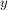is an integer, and 3 is the remainder when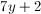is divided by 5, then which of the following is a possible value of? Possible Answers: 8 1 2 5 9 Correct answer: 8 Explanation: For this problem, you need to use process of elimination. When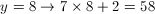The remainder of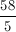is 3 (the answer is 11, remainder 3), sois a possible answer choice. If you try the other answers they won't work. Example Question #1 : Operations The annual number of cellular phones purchased from a company is illustrated in the following graph. How many more phones were sold in 2009 than 2011?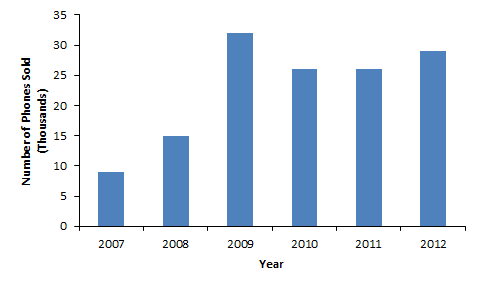Possible Answers: 17 6,000 6 3,000 17,000 Correct answer: 6,000 Explanation: In 2009, 32,000 phones were sold (according the the label on the y-axis, each number is equal to 1,000 phones). In 2011, 26,000 phones were sold.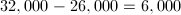Example Question #2 : Operations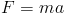in physics where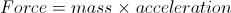. If the mass is increased by 3 three times the original and the acceleration is increased by 7 times the original, how many times greater is the new force than the original force? Possible Answers: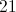Correct answer:Explanation: We simply multiply the new mass by the new acceleration to obtain a new force that is 21 times greater than the original. Example Question #3 : Operations An office wants to buy 22 computers at$900 each. The budget is $20,000 and the tax on computers is 9%. How many computers can the office afford? Possible Answers:Correct answer:Explanation: The office can only afford 20 computers. 1.09 * 900 =$981 is the actual price of each computer, with tax.

Divide:

20,000/981 = 20.39

Since the office cannot purchase a partial computer, we round down to 20 computers.

Example Question #4 : Operations

A paint crew can paintwalls inhours. At this rate, how many walls can the paint crew paint inhours?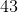Explanation:

First, dividebyto determine how many walls the paint crew can paint inhour.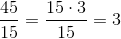divided byis equal to.

Then, multiply this number byhours.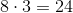Example Question #1 : New Sat Math Calculator

Convert three yards to inches.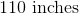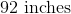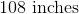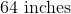Explanation:

To solve this problem, we need to know the conversions between yards to feet, and feet to inches.  Write their correct conversions.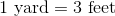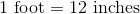Convert three yards to feet.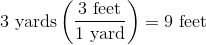Convert nine feet to inches.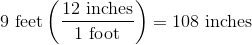← Previous 1